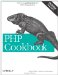# Recipe 5.1. AvoidingVersusConfusion

### Recipe 5.1. Avoiding == Versus = Confusion

#### 5.1.1. Problem

You don't want to accidentally assign values when comparing a variable and a constant.

#### 5.1.2. Solution

Use:

`if (12 == \$dwarves) { ... }`

`if (\$dwarves == 12) { ... }`

Putting the constant on the left triggers a parse error with the assignment operator. In other words, PHP complains when you write:

`if (12 = \$dwarves) { ... }`

but:

`if (\$dwarves = 12) { ... }`

silently executes, assigning 12 to the variable \$dwarves, and then executing the code inside the block. (\$dwarves = 12 evaluates to 12, which is true.)

#### 5.1.3. Discussion

Putting a constant on the left side of a comparison coerces the comparison to the type of the constant. This causes problems when you are comparing an integer with a variable that could be an integer or a string. 0 == \$dwarves is true when \$dwarves is 0, but it's also true when \$dwarves is sleepy. Since an integer (0) is on the left side of the comparison, PHP converts what's on the right (the string sleepy) to an integer (0) before comparing. To avoid this, use the identity operator, 0 === \$dwarves, instead.

Documentation for = at http://www.php.net/language.operators.assignment.php and for == and === at http://www.php.net/manual/language.operators.comparison.php.PHP Cookbook: Solutions and Examples for PHP Programmers
ISBN: 0596101015
EAN: 2147483647
Year: 2006
Pages: 445

Similar book on Amazon